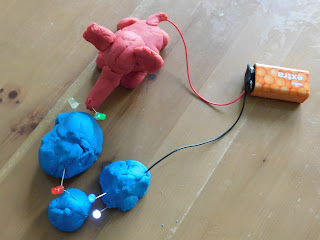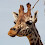### Electric play dough - a bit more explanation

A friend and her daughter tried our electric play dough activity and enjoyed it.  However, she gave me some helpful feedback - they would have found it easier had there been a bit more explanation of the science behind it so my friend (who isn't a scientist) would have been able to better predict what would and wouldn't work.  If you read this, thanks for the helpful comment!

Here's an attempt at a bit of further explanation for electric play dough circuits, but I've not studied anything to do with electricity for a long time, so if anyone can improve it then please do leave a comment!

It's not at all designed to be the explanation to give a small child, but to assist grown ups with a hazy memory to be more confident in trying the activity with children.

What is voltage?
You can think of this as the 'push' of electrons around a circuit, such that a greater voltage means a battery is more able to 'push' the electricity around.  More properly, its the difference in electrical potential energy between two points.  You measure voltage in units called Volts (abbreviated to V).

What is current?
You can think of this as the 'flow' of electrons around a circuit, such that a higher current means more electricity is flowing.  The flow of electrons needs to be around a complete circuit, i.e. there needs to be a route from one terminal of the battery to the other.  Current is the amount of charge flowing per unit time and is measured in a unit called Amperes, commonly referred to as Amps (abbreviated to A).

What is resistance?
This how difficult it is for the charge to flow around a circuit, i.e. how much something reduces the current flowing.  Electrical resistance is measured in something called Ohms (abbreviated to Ω).

An analogy
If you think of a container of water with a pipe coming out of the bottom, the charge is analogous to the amount of water in the container, and the voltage analogous to the water pressure in the pipe.  So the more water in your container, the greater the pressure in the pipe at the bottom.  Taking this analogy, the current is the amount of water flowing through the pipe.  If you have a higher voltage (pressure of water), then the current (amount of water flowing) is higher.

If you had two identical water containers as above, but one had a wider pipe than the other, the water would flow faster out of that one i.e. the resistance is lower and the current (flow rate) is higher.  If you want the water in the narrower pipe to flow at the same rate, you'd need more water in the container to increase the water pressure; similarly if you want to increase the current in an electrical circuit with more resistance then you need to increase the voltage.

Ohm's law
You might remember this one from school, Ohm's law can be expressed as the formula V=I.R (where I is current).  In other words, voltage = current x resistance.  If you rearrange this, you can see that current = voltage / resistance, or resistance = voltage / current, and this lets you work out what will happen e.g. if you increase the voltage or the resistance in your circuit.

So what's this got to do with electric play dough?
LEDs need sufficient current (in the right direction) to make them light.  To get enough current in the electric play dough I blogged about, you need a 9 Volt battery.  This is because play dough is a poor conductor compared to a copper wire, so it's got a lot of resistance.  To get enough current, you need more voltage than a 1.5 Volt battery (e.g. an AA) can provide; when I tried one LED with two lumps of play dough at each end of the AA battery, there wasn't even a faint glow from the LED.

You shouldn't put the LEDs straight across the 9V battery terminals because this causes too much current flows through the LED and it will burn out after lighting brightly for a while.  Without the resistance provided by the play dough, the current is too high.

You need a complete circuit for the current to flow and the LEDs to light.  This is why when we made traffic lights, the spoon acted as a switch in completing a circuit that contained one (or two) of the LEDs.  You also need to force the current to flow through the LED by leaving a gap between the play dough - if you just stick both ends of the LED into one blob of play dough and connect it to a battery, the current doesn't pass through the LED and there's no light.

Our circuit with 5 LEDs in series (in a line one after the other in the circuit) didn't work i.e. none of the LEDs lit.  This can be explained by the lack of current.  There's too much resistance in the circuit in total, so the current flowing around the circuit is just too low to make the LEDs emit light.  If you take out some of the lumps of play dough (which add resistance) and some of the LEDs, the current increases.  With more current, the LEDs glow more brightly.

In the simple parallel circuits we made, the current flowing through each LED is higher than if the same number of LEDs were in series.  The LEDs therefore light more brightly in parallel than in series.  You can think about this from the perspective of voltage - the difference in charge across each LED (the voltage) is higher than for the LEDs in the series circuit so the current is greater.  If you want more LEDs in your electric play dough, the trick is therefore to make at least some of them in parallel.  We managed 5 in parallel when we were making circuits with cutlery.  We've built a few circuits where the LEDs are a mixture of series and parallel - in the one below, the LEDs lit nicely!Our most recent play dough circuit - an elephant holding parallel LEDs with its trunk!

[Edited on 1/9/19 to take account of comment below re: definition of voltage]

1.Hello from the electric play dough friend's husband! :-) I've been following your blog with interest, and not least am very pleased to know what an ossicone is!

Being pedantic, I'm afraid voltage isn't the difference in *charge* between 2 points but the difference in electrical potential energy (hence 1 Volt is 1 Joule per Coulomb). Sorry...

Also, as well as the resistance reason for LEDs not lighting with AA batteries (or lots in series on a 9V battery), there's the fact that they each have a minimum voltage they need to see across them before they will light up - which is related to the wavelength of the colour they emit by E=hf, hence red LEDs will light at a lower voltage than blue ones. If there is enough voltage but high resistance then you'll often see them lighting up really dimly. But please don't ask me as a lowly engineer to explain anything quantum!

We hope to catch up with you all again soon.

1.Thanks for the clarification, you are of course correct on voltage. I blame too little sleep in recent months! I will edit above when I get a chance!

2.Thanks for a very clear explanation for a non-scientist :). It's always good to learn something new!